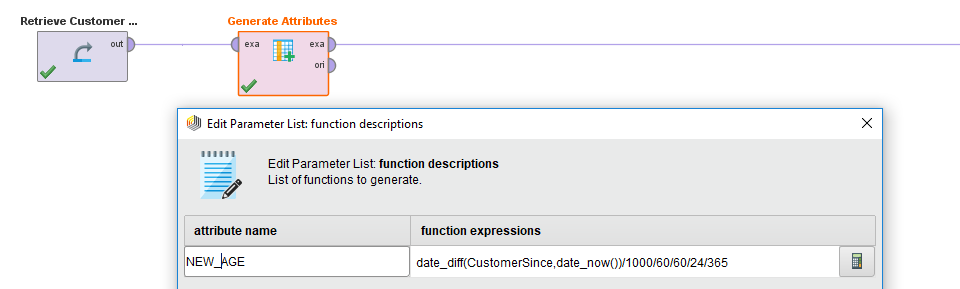# Age

Member Posts: 1Newbie
Hi! I am trying to create an atrribute which contains the age of a person since de day of birth to Agust 8 1990. Does anybody know how to do it?

• Employee, RapidMiner Certified Analyst, RapidMiner Certified Expert, Member Posts: 220RM Data Scientist
edited September 5
Hi @paolamzavarce

"Generate Attribute" operator is your best friendYou can use date_diff() function in the formula to calculate the gap between the birthday and Aug 8 1990. Just make sure date_diff is applied to two dates. And it will return the time difference in milliseconds. You can convert into years by rescaling.

Cheers,
YY

• Employee, RapidMiner Certified Analyst, RapidMiner Certified Expert, Member Posts: 220RM Data Scientist```<?xml version="1.0" encoding="UTF-8"?><process version="9.3.001">
<context>
<input/>
<output/>
<macros/>
</context>
<operator activated="true" class="process" compatibility="9.3.001" expanded="true" name="Process">
<parameter key="logverbosity" value="init"/>
<parameter key="random_seed" value="2001"/>
<parameter key="send_mail" value="never"/>
<parameter key="notification_email" value="[email protected]"/>
<parameter key="process_duration_for_mail" value="1"/>
<parameter key="encoding" value="SYSTEM"/>
<process expanded="true">
<operator activated="true" class="retrieve" compatibility="9.3.001" expanded="true" height="68" name="Retrieve Customer Data" width="90" x="112" y="34">
<parameter key="repository_entry" value="//Samples/Templates/Churn Modeling/Customer Data"/>
</operator>
<operator activated="true" class="generate_attributes" compatibility="9.3.001" expanded="true" height="82" name="Generate Attributes" width="90" x="313" y="34">
<list key="function_descriptions">
<parameter key="NEW_AGE" value="date_diff(CustomerSince,date_now())/1000/60/60/24/365"/>
</list>
<parameter key="keep_all" value="true"/>
</operator>
<connect from_op="Retrieve Customer Data" from_port="output" to_op="Generate Attributes" to_port="example set input"/>
<connect from_op="Generate Attributes" from_port="example set output" to_port="result 1"/>
<portSpacing port="source_input 1" spacing="0"/>
<portSpacing port="sink_result 1" spacing="0"/>
<portSpacing port="sink_result 2" spacing="0"/>
</process>
</operator>
</process>
```